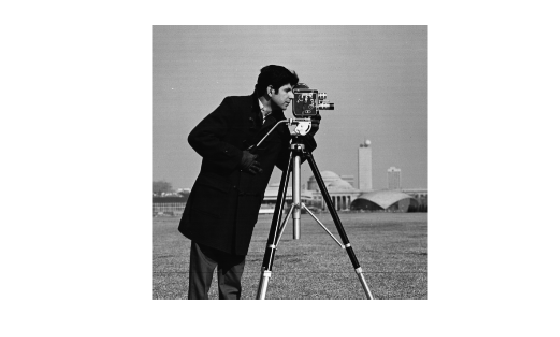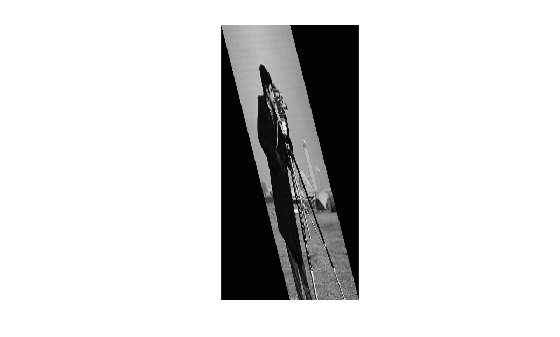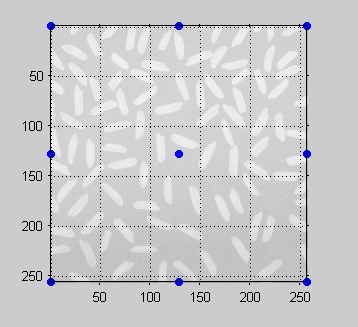findbounds

Find output bounds for spatial transformation

Description

example

outbounds = findbounds(tform,inbounds) estimates the output bounds corresponding to a given spatial transformation and a set of input bounds. tform is a spatial transformation structure. inbounds is a 2-by-num_dims matrix that specifies the lower and upper bounds of the output image. outbounds is an estimate of the smallest rectangular region completely containing the transformed rectangle represented by the input bounds, and has the same form as inbounds. Since outbounds is only an estimate, it might not completely contain the transformed input rectangle.

Examples

collapse all

Read an image into the workspace, and display the image.

figure
imshow(I)Create a spatial transformation structure that stretches an image.

T = maketform('affine',[.5 0 0; .5 2 0; 0 0 1]);

Calculate the boundaries of the output image, given the size of the input image and the spatial transformation. The dimensions of the input image are 256-by-256. The boundaries of the output image reflect the transformation: 256-by-512.

outb = findbounds(T,[0 0;256 256])
outb = 2×2

0     0
256   512

Apply the transformation, and display the image.

transformedI = imtransform(I,T);
figure
imshow(transformedI)Input Arguments

collapse all

Spatial transformation, specified as a structure (tform).

Data Types: struct

Bounds for each dimension of the input image, specified as a 2-by-num_dims matrix. The first row of inbounds specifies the lower bounds for each dimension, and the second row specifies the upper bounds. num_dims has to be consistent with the ndims_in field of tform.

Example: outb = findbounds(T,[0 0;256 256]) where input image is 256-by-256.

Data Types: double

Output Arguments

collapse all

Bounds for each dimension of the output image (output space bounding box), returned as a 2-by-num_dims matrix of class double.

Algorithms

1. findbounds first creates a grid of input-space points. These points are at the center, corners, and middle of each edge in the image.

h = imshow(I);
axis on, grid on
in_points = [ ...
0.5000    0.5000
0.5000  256.5000
256.5000    0.5000
256.5000  256.5000
0.5000  128.5000
128.5000    0.5000
128.5000  128.5000
128.5000  256.5000
256.5000  128.5000];
hold on
plot(in_points(:,1),in_points(:,2),'.','MarkerSize',18)
hold off

Grid of Input-Space Points2. Next, findbounds transforms the grid of input-space points to output space. If tform contains a forward transformation (a nonempty forward_fcn field), then findbounds transforms the input-space points using tformfwd. For example:

tform = maketform('affine', ...
[1.1067 -0.2341 0; 0.5872 1.1769 0; 1000 -300 1]);
out_points = tformfwd(tform, in_points)
out_points =

1.0e+03 *

1.0008   -0.2995
1.1512    0.0018
1.2842   -0.3595
1.4345   -0.0582
1.0760   -0.1489
1.1425   -0.3295
1.2177   -0.1789
1.2928   -0.0282

If tform does not contain a forward transformation, then findbounds estimates the output bounds using the Nelder-Mead optimization function fminsearch.

3. Finally, findbounds computes the bounding box of the transformed grid of points.